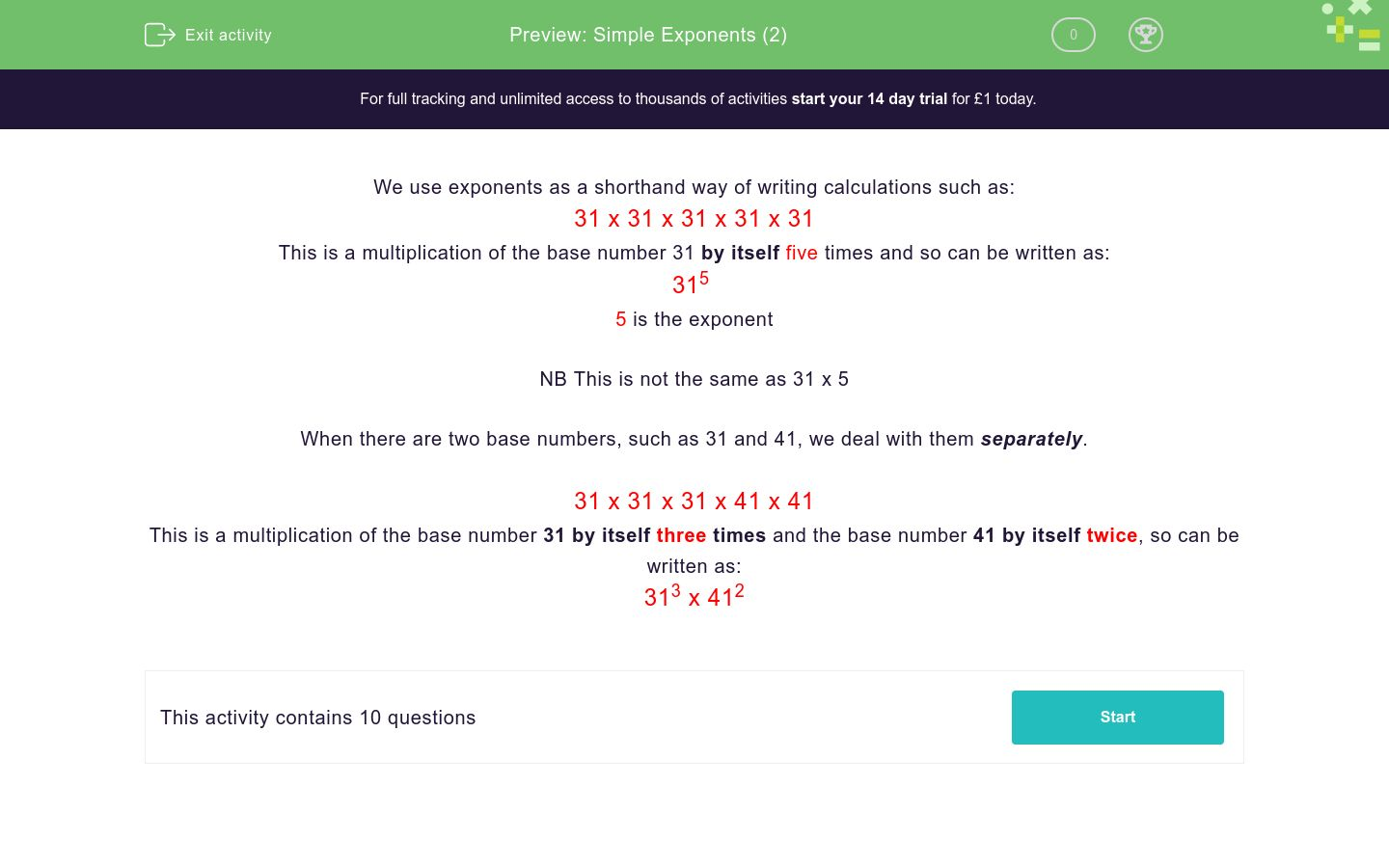# Simple Exponents (2)

In this worksheet, students understand and use simple exponents.Key stage:  KS 3

Curriculum topic:   Number

Curriculum subtopic:   Understand Integer Powers/Real Roots

Difficulty level:### QUESTION 1 of 10

We use exponents as a shorthand way of writing calculations such as:

31 x 31 x 31 x 31 x 31

This is a multiplication of the base number 31 by itself five times and so can be written as:

315

5 is the exponent

NB This is not the same as 31 x 5

When there are two base numbers, such as 31 and 41, we deal with them separately.

31 x 31 x 31 x 41 x 41

This is a multiplication of the base number 31 by itself three times and the base number 41 by itself twice, so can be written as:

313 x 412

What are the exponents, m and n, when we write the following as 14m x 23n:

14 x 14 x 14 x 23 x 23

## Column B

m
2
n
3

What are the exponents, m and n, when we write the following as 14m x 23n:

14 x 14 x 14 x 14 x 23 x 23

## Column B

m
4
n
2

What are the exponents, m and n, when we write the following as 14m x 23n:

14 x 14 x 14 x 14 x 23 x 23 x 23

## Column B

m
3
n
4

What are the exponents, m and n, when we write the following as 14m x 23n:

14 x 14 x 14 x 14 x 23 x 23 x 23 x 23 x 23

## Column B

m
4
n
5

What are the exponents, m and n, when we write the following as 14m x 23n:

14 x 14 x 14 x 23 x 23 x 23 x 23 x 23

## Column B

m
3
n
5

What are the exponents, m and n, when we write the following as 14m x 23n:

14 x 23 x 14 x 23 x 23 x 23 x 23 x 23

## Column B

m
2
n
6

What are the exponents, m and n, when we write the following as 14m x 23n:

14 x 23 x 14 x 23 x 14 x 14 x 23

## Column B

m
4
n
3

What are the exponents, m and n, when we write the following as 4m x 3n:

4 x 3 x 4 x 3 x 4 x 4 x 3 x 4

## Column B

m
3
n
5

What are the exponents, m and n, when we write the following as 4m x 3n:

4 x 4 x 4 x 3 x 4 x 4 x 3 x 4

## Column B

m
6
n
2

What are the exponents, m and n, when we write the following as 40m x 30n:

40 x 40 x 40 x 30 x 40 x 40 x 30 x 40

## Column B

m
6
n
2
• Question 1

What are the exponents, m and n, when we write the following as 14m x 23n:

14 x 14 x 14 x 23 x 23

## Column B

m
3
n
2
• Question 2

What are the exponents, m and n, when we write the following as 14m x 23n:

14 x 14 x 14 x 14 x 23 x 23

## Column B

m
4
n
2
• Question 3

What are the exponents, m and n, when we write the following as 14m x 23n:

14 x 14 x 14 x 14 x 23 x 23 x 23

## Column B

m
4
n
3
• Question 4

What are the exponents, m and n, when we write the following as 14m x 23n:

14 x 14 x 14 x 14 x 23 x 23 x 23 x 23 x 23

## Column B

m
4
n
5
• Question 5

What are the exponents, m and n, when we write the following as 14m x 23n:

14 x 14 x 14 x 23 x 23 x 23 x 23 x 23

## Column B

m
3
n
5
• Question 6

What are the exponents, m and n, when we write the following as 14m x 23n:

14 x 23 x 14 x 23 x 23 x 23 x 23 x 23

## Column B

m
2
n
6
• Question 7

What are the exponents, m and n, when we write the following as 14m x 23n:

14 x 23 x 14 x 23 x 14 x 14 x 23

## Column B

m
4
n
3
• Question 8

What are the exponents, m and n, when we write the following as 4m x 3n:

4 x 3 x 4 x 3 x 4 x 4 x 3 x 4

## Column B

m
5
n
3
• Question 9

What are the exponents, m and n, when we write the following as 4m x 3n:

4 x 4 x 4 x 3 x 4 x 4 x 3 x 4

## Column B

m
6
n
2
• Question 10

What are the exponents, m and n, when we write the following as 40m x 30n:

40 x 40 x 40 x 30 x 40 x 40 x 30 x 40

## Column B

m
6
n
2
---- OR ----

Sign up for a £1 trial so you can track and measure your child's progress on this activity.

### What is EdPlace?

We're your National Curriculum aligned online education content provider helping each child succeed in English, maths and science from year 1 to GCSE. With an EdPlace account you’ll be able to track and measure progress, helping each child achieve their best. We build confidence and attainment by personalising each child’s learning at a level that suits them.

Get started